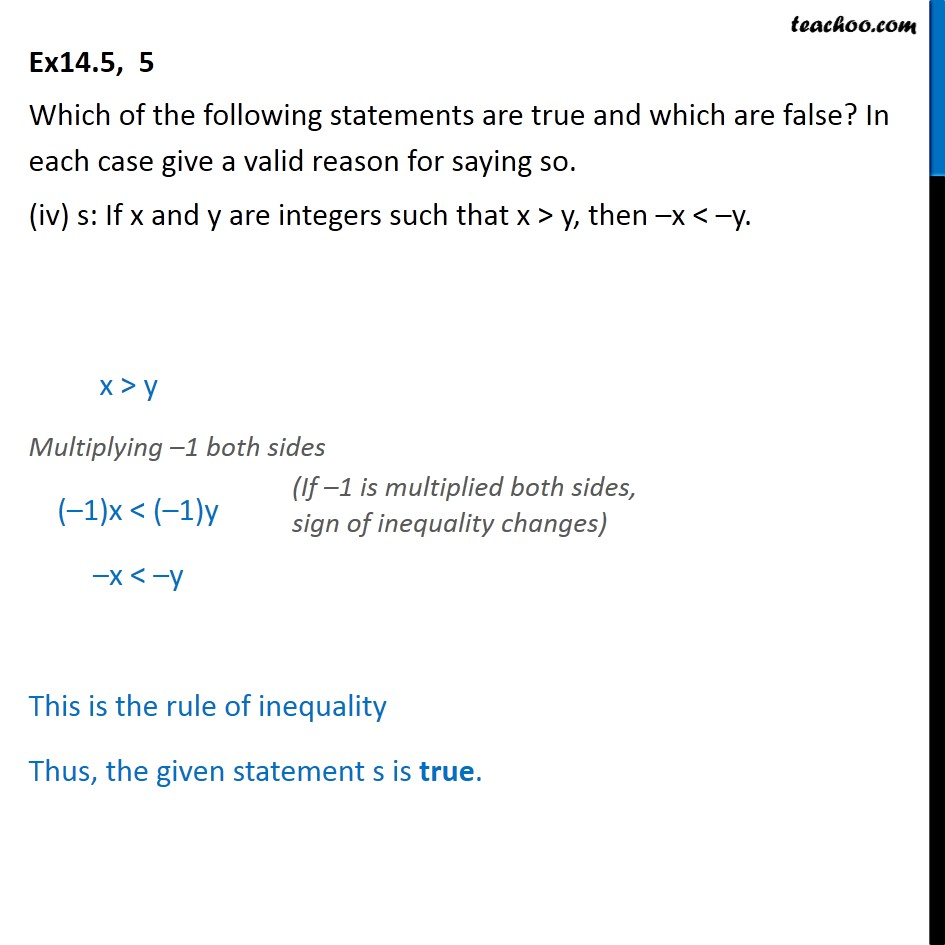Proving Statements true or false

Mathematical Reasoning
Serial order wiseLearn in your speed, with individual attention - Teachoo Maths 1-on-1 Class

### Transcript

Ex14.5, 5 Which of the following statements are true and which are false? In each case give a valid reason for saying so. (iv) s: If x and y are integers such that x > y, then –x < –y. x > y Multiplying –1 both sides (–1)x < (–1)y –x < –y This is the rule of inequality Thus, the given statement s is true.